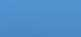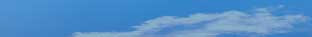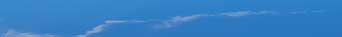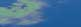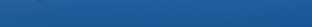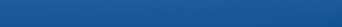Freeware, shareware, software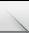AuthorsFeedbackSite SearchSoftware Categories# STFMath 1.1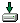STFMath, technically enhanced math utility, allows you to: draw Cartesian (standard or parametric) and Polar functions, special functions (Gamma, Beta, Fourier, Blancmange) or Integral (with Average preview) in MDI (Multi Documents Interface) supported Graph Mode (open up to 10 independent graph documents in the same window). Supported functions list (logarithm, trigonometric, exponential, hyperbolic) is extended with predefined functions. • In the same window it is possible to draw unlimited number of functions, with different attributes (grid scaling, center moving, graph colors, textual labels inserted etc.). Additional options are: • Calculate integral, derivative and average values, and draw integrals with average preview • Calculate and draw tangent and normal and trace drawn functions • Perform function analyze (calculate roots, critical points, Y or two functions intersection), calculate table of values and publish it as HTML • Evaluate any function or perform various additional calculations with built-in scientific, complex and geometry (with units converter) calculators • Save drawn graph as an image (.BMP, .WMF, .JPG), copy it to clipboard or print graphs and graph history • Build presentation with MathPad, fully functional text editor. • Use scientific, geometry, complex, geometry unit converter. • Use matrix (up to 50x50 in size, with complex numbers support) calculator with supported operations: - Matrix addition, subtraction, multiplication, scalar multiplication and division; - Calculation of matrix determinant and trace, characteristic equation (for 2x2 and 3x3 matrix), adjoint (adjugate) and inverse matrix; - Matrix edition (swap matrixes, swap and delete rows or columns, multiply and submit rows and columns, transpose matrix and more.
 Version: 1.1 Release Date: 11/30/-1 Size: 5450 KB Platform: Win95,Win98,WinXP,Windows2000 Program type:  Shareware Cost: \$24 Online order: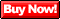Review: Click here
 System requirements: Win9x/XPno serial numbers, keygen (key generators), cracks, patches can be found here   © 2007 DrCreator.com & Webmedia.Ru